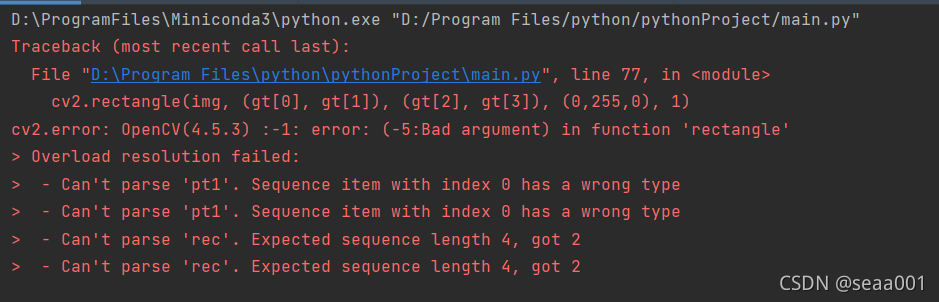# Opencv453 drawing rectangle function error reporting solution

``````# draw gt
cv2.rectangle(img, (gt, gt), (gt, gt), (0,255,0), 1)``````

The following error occurred while running:The rectangle function in opencv453 is incompatible with floating-point data

terms of settlement:

It is OK to convert the two coordinate data types in the rectangle function to int

``cv2.rectangle(img, (int(gt), int(gt)), (int(gt), int(gt)),(0,255,0), 1)``